# Bellman-Ford Algorithm in C and C++

Here you will learn about Bellman-Ford Algorithm in C and C++.

Dijkstra and Bellman-Ford Algorithms used to find out single source shortest paths. i.e. there is a source node, from that node we have to find shortest distance to every other node. Dijkstra algorithm fails when graph has negative weight cycle. But Bellman-Ford Algorithm won’t fail even, the graph has negative edge cycle. If there any negative edge cycle it will detect and say there is negative edge cycle. If not it will give answer to given problem.

Bellman-Ford Algorithm will work on logic that, if graph has n nodes, then shortest path never contain more than n-1 edges. This is exactly what Bellman-Ford do. It is enough to relax each edge (v-1) times to find shortest path. But to find whether there is negative cycle or not we again do one more relaxation. If we get less distance in nth relaxation we can say that there is negative edge cycle. Reason for this is negative value added and distance get reduced.

Relaxing edge

In algorithm and code below we use this term Relaxing edge.

Relaxing edge is an operation performed on an edge (u, v) . when,

d(u) > d(v) + Cost(u,v)

Here d(u) means distance of u. If already known distance to “u” is greater than the path from “s” to “v” and “v” to “u” we update that d(u) value with d(v) + cost(u,v).

### Algorithm and Time Complexity

Finally time complexity is (v-1) (E) O(1) = O(VE)

### Example Problem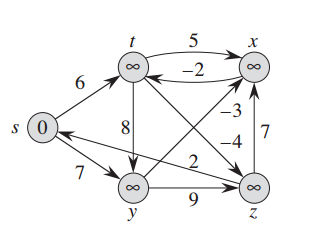This is the given directed graph.

(s,t) = 6  (y,x) = -3

(s,y)= 7  (y,z) = 9

(t,y) = 8  (x,t) = -2

(t,z) = -4  (z,x) = 7

(t,x) = 5  (z,s) = 2

Above we can see using vertex “S” as source (Setting distance as 0), we initialize all other distance as infinity.

 S T X Y Z distance 0 ∞ ∞ ∞ ∞ Path – – – – –

Table and Image explanation: This table, 2nd row shows distance from source to that particular node ad 3rd row shows to reach that node what is the node we visited recently. This path we can see in the image also.

Note: In each iteration, iteration “n” means it contains the path at most “n” edges. And while we are doing iteration “n” we must follow the graph which we obtained in iteration “n-1”.

Iteration 1: edge (s,t) and (z,y) relaxed and updating the distances to t and y.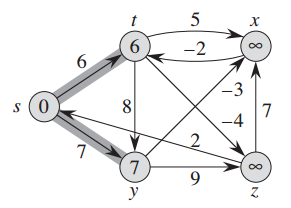S T X Y Z distance 0 6 ∞ 7 ∞ Path – S – S –

Iteration 2 : edge (t,z) and (y,x) relaxed and x and z values are updated.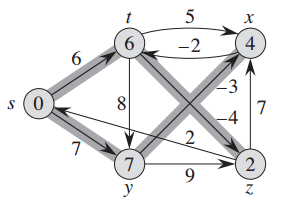S T X Y Z distance 0 6 4 7 2 Path – S Y S T

Iteration 3: Value of t updated by relaxing (x,t)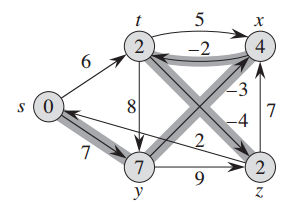S T X Y Z distance 0 2 4 7 2 Path – X Y S T

Iteration 4: Value of z updated by relaxing edge (t,z)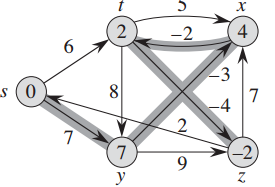Until now 4 iterations completed and shortest path found to every node form source node. Now we have to do one more iteration to find whether there exists negative edge cycle or not. When we do this nth (5th here) relaxation if we found less distance to any vertex from any other path we can say that there is negative edge cycle. Here we can relax any edge to graph which obtained in iteration 4and we can observe that there is no chance to change those values. So we can confirm that there is no negative edge cycle in this graph.

## Program for Bellman-Ford Algorithm in C

### Code explanation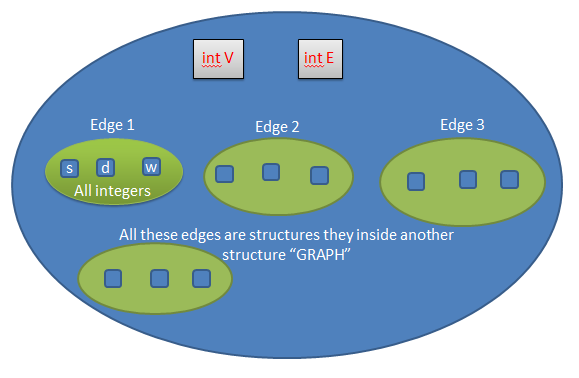This picture shows the Structure of our input graph.

We create a structure called “Graph” which contains two integers int v (represent number of vertices) and int E (represents number of edges) and also another structure inside this structure which represents edge. That structure contains 3 integers source, destination, weight of that edge. So we create “E” number of structures inside the structure Graph.

After creating Graph, choose a source node and send to BellmanFord function. In this function we relax each edge “v-1” times. After this we can store the result of shortest paths in an array. And we do one more relaxation to find whether there exists negative edge cycle or not. If we got less distance at any node in Vth relaxation of edges, then we can say that the graph have negative edge cycle.

Output

Enter number of vertices in graph
5
Enter number of edges in graph
10
0

Enter edge 1 properties Source, destination, weight respectively
0 1 6

Enter edge 2 properties Source, destination, weight respectively
0 2 7

Enter edge 3 properties Source, destination, weight respectively
1 2 8

Enter edge 4 properties Source, destination, weight respectively
1 4 -4

Enter edge 5 properties Source, destination, weight respectively
1 3 5

Enter edge 6 properties Source, destination, weight respectively
3 1 -2

Enter edge 7 properties Source, destination, weight respectively
2 3 -3

Enter edge 8 properties Source, destination, weight respectively
2 4 9

Enter edge 9 properties Source, destination, weight respectively
4 0 2

Enter edge 10 properties Source, destination, weight respectively
4 3 7

Vertex  Distance from Source Vertex
0  0
1  2
2  7
3  4
4  -2

## Program for Bellman-Ford Algorithm in C++

Reference: Introduction to Algorithms by Thomas H. Cormen

Comment below if you have queries or found anything incorrect in above tutorial for Bellman-Ford Algorithm in C and C++.

### 5 thoughts on “Bellman-Ford Algorithm in C and C++”

1.These Algorithms are quite complex, maybe I need study and learn more to understand. Thanks for sharing the article.

2.your algorith is nice very helful to me during my preparation time and you code nicely

3.Your program is wrong. Before relaxing you should check if u vertex of u-v pair is not set to INT_MAX.

4.code copy ka button bhi laga deta bhai sath hi

5.i tried it with a different slightly bigger graph, it failed. Though it did work for small problems.
i dont know where the problem is but thought you should be aware.